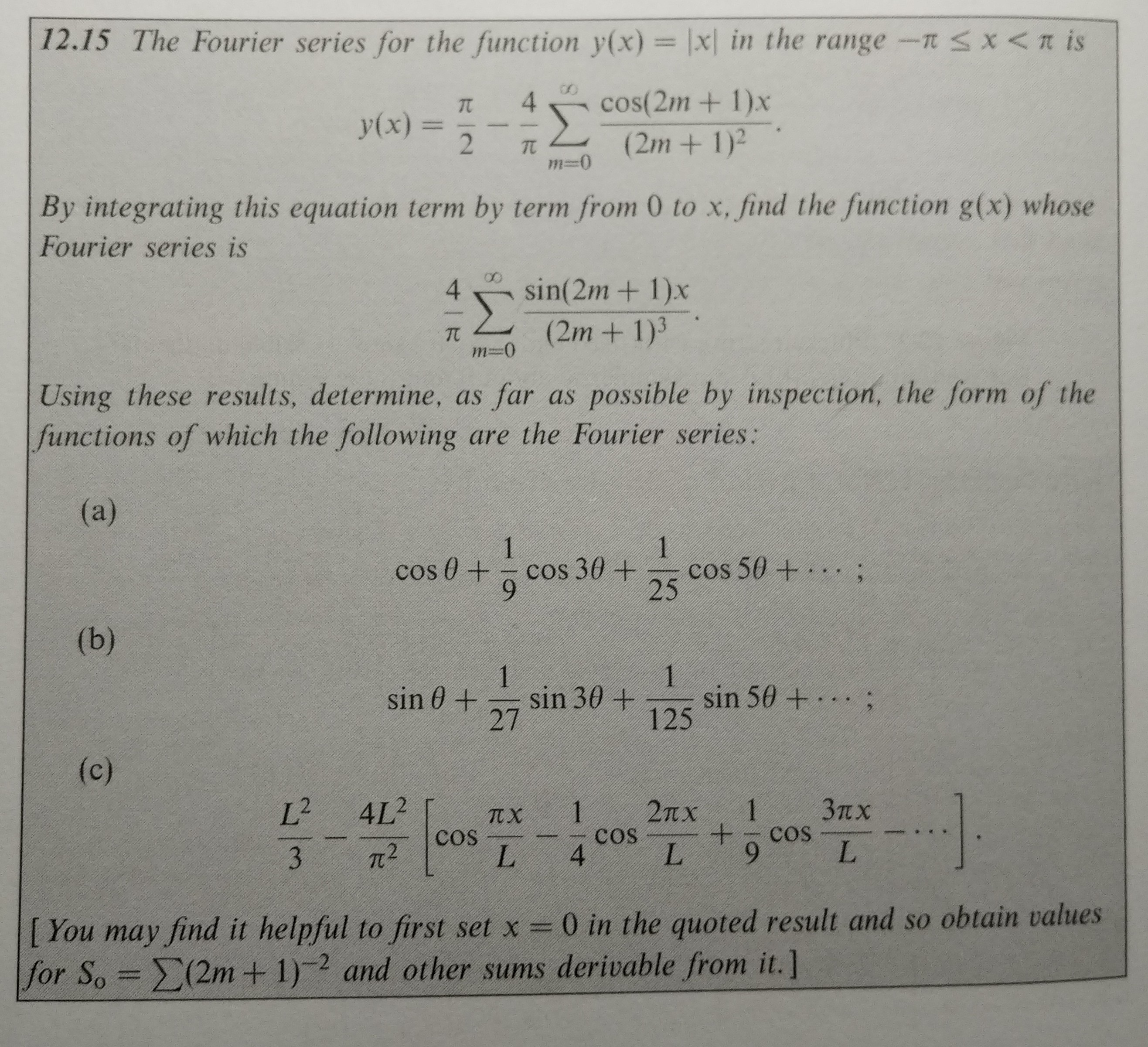# 12.15 The Fourier series for the function y(x) = \x( in the range-mx < π is4cos(2m + 1)xy(x) = 2-π(2m + 1)2By integrating this equation term by term from 0 to x, find the function g(x) whoseFourier series is4 sin(2m+ 1)x2m + 1)-0 (InUsing these results, determine, as far as possible by inspection, the form of thefunctions of which the following are the Fourier series:cos θ + 0 cos 3 θ +cos 504 . . . ;2527125し、9I You may find it helpful to first set x-0 in the quoted result and so obtain valuesfor S.-Σ(2m + 1)-2 and other sums derivable from it.]

Question
48 viewshelp_outlineImage Transcriptionclose12.15 The Fourier series for the function y(x) = \x( in the range-m x < π is 4cos(2m + 1)x y(x) = 2-π (2m + 1)2 By integrating this equation term by term from 0 to x, find the function g(x) whose Fourier series is 4 sin(2m+ 1)x 2m + 1) -0 (In Using these results, determine, as far as possible by inspection, the form of the functions of which the following are the Fourier series: cos θ + 0 cos 3 θ + cos 504 . . . ; 25 27 125 し、9 I You may find it helpful to first set x-0 in the quoted result and so obtain values for S.-Σ(2m + 1)-2 and other sums derivable from it.] fullscreen
check_circle

Step 1

To determine the related functions and the Fourier series based on the given Fourier series

Step 2

Carry out the steps as shown.

Step 3

Observe that we have a sine series on the right hand side of the equation (2). So we extend the function (the one obtain...

### Want to see the full answer?

See Solution

#### Want to see this answer and more?

Solutions are written by subject experts who are available 24/7. Questions are typically answered within 1 hour.*

See Solution
*Response times may vary by subject and question.
Tagged in

### Math